# Recurrent Neural Networks¶

Sentiment Analysis is the problem of identifying the writer's sentiment given a piece of text. Sentiment Analysis can be applied to movie reviews, feedback of other forms, emails, tweets, course evaluations, and much more.

Rudimentary forms of sentiment analysis might involve scoring each word on a scale from "sad" to "happy", then averaging the "happiness score" of each word in a piece of text. This technique has obvious drawbacks: it won't be able to handle negation, sarcasm, or any complex syntactical form. We can do better.

In this demonstration, we will use a recurrent neural network to classify the sentiment of a piece of text (a sequence of words). We'll use GloVe embeddings as inputs to the recurrent network.

As a sidenote, not all recurrent neural networks use word embeddings as input. If we had a small enough vocabulary, we could have used a one-hot embedding of the words.)

## Data¶

We'll focus on the problem of classifying tweets as having positive or negative emotions. We use the Sentiment140 data set, which contains tweets with either a positive or negative emoticon. Our goal is to determine whether which type of emoticon the tweet (with the emoticon removed) contained. The dataset was actually collected by a group of students, much like you, who are doing their first machine learning projects.

Let's look at the data:

In :
import csv
import torch
import torch.nn as nn
import torch.nn.functional as F
import torch.optim as optim
import torchtext
import numpy as np
import matplotlib.pyplot as plt

def get_data():
# This is a very large file, so we will not load it into RAM
return csv.reader(open("data/training.1600000.processed.noemoticon.csv", "rt", encoding="latin-1"))

for i, line in enumerate(get_data()):
if i > 10:
break
print(line)

['0', '1467810369', 'Mon Apr 06 22:19:45 PDT 2009', 'NO_QUERY', '_TheSpecialOne_', "@switchfoot http://twitpic.com/2y1zl - Awww, that's a bummer.  You shoulda got David Carr of Third Day to do it. ;D"]
['0', '1467810672', 'Mon Apr 06 22:19:49 PDT 2009', 'NO_QUERY', 'scotthamilton', "is upset that he can't update his Facebook by texting it... and might cry as a result  School today also. Blah!"]
['0', '1467810917', 'Mon Apr 06 22:19:53 PDT 2009', 'NO_QUERY', 'mattycus', '@Kenichan I dived many times for the ball. Managed to save 50%  The rest go out of bounds']
['0', '1467811184', 'Mon Apr 06 22:19:57 PDT 2009', 'NO_QUERY', 'ElleCTF', 'my whole body feels itchy and like its on fire ']
['0', '1467811193', 'Mon Apr 06 22:19:57 PDT 2009', 'NO_QUERY', 'Karoli', "@nationwideclass no, it's not behaving at all. i'm mad. why am i here? because I can't see you all over there. "]
['0', '1467811372', 'Mon Apr 06 22:20:00 PDT 2009', 'NO_QUERY', 'joy_wolf', '@Kwesidei not the whole crew ']
['0', '1467811592', 'Mon Apr 06 22:20:03 PDT 2009', 'NO_QUERY', 'mybirch', 'Need a hug ']
['0', '1467811594', 'Mon Apr 06 22:20:03 PDT 2009', 'NO_QUERY', 'coZZ', "@LOLTrish hey  long time no see! Yes.. Rains a bit ,only a bit  LOL , I'm fine thanks , how's you ?"]
['0', '1467811795', 'Mon Apr 06 22:20:05 PDT 2009', 'NO_QUERY', '2Hood4Hollywood', "@Tatiana_K nope they didn't have it "]
['0', '1467812025', 'Mon Apr 06 22:20:09 PDT 2009', 'NO_QUERY', 'mimismo', '@twittera que me muera ? ']
['0', '1467812416', 'Mon Apr 06 22:20:16 PDT 2009', 'NO_QUERY', 'erinx3leannexo', "spring break in plain city... it's snowing "]


The columns we care about is the first one and the last one. The first column is the label (the label 0 means "sad" tweet, 4 means "happy" tweet), and the last column contains the tweet. Our task is to predict the sentiment of the tweet given the text.

We will need to split the text into words. We will do so by splitting at all whitespace characters. There are better ways to perform the split, but let's keep our dependencies light.

In :
def split_tweet(tweet):
# separate punctuations
tweet = tweet.replace(".", " . ") \
.replace(",", " , ") \
.replace(";", " ; ") \
.replace("?", " ? ")
return tweet.lower().split()


Since tweets often have mispellings, we'll need to ignore words that do not appear in the Glove embeddings. Let's sanity check that there are enough words for us to work with.

In :
import torchtext
glove = torchtext.vocab.GloVe(name="6B", dim=50)

for i, line in enumerate(get_data()):
if i > 30:
break
print(sum(int(w in glove.stoi) for w in split_tweet(line[-1])))

21
23
17
10
22
4
3
21
4
3
9
4
19
15
19
18
18
4
9
13
11
23
8
9
4
11
13
6
23
20
13


Looks like each tweet has at least one word that has an embedding.

## Training Data¶

We will only use $\frac{1}{29}$ of the data in the file, so that this demo runs relatively quickly.

Since we are going to store the individual words in a tweet, we will defer looking up the word embeddings. Instead, we will store the index of each word in a PyTorch tensor. Our choice is the most memory-efficient, since it takes fewer bits to store an integer index than a 50-dimensional vector or a word.

In :
def get_tweet_words(glove_vector):
train, valid, test = [], [], []
for i, line in enumerate(get_data()):
if i % 29 == 0:
tweet = line[-1]
idxs = [glove_vector.stoi[w]        # lookup the index of word
for w in split_tweet(tweet)
if w in glove_vector.stoi] # keep words that has an embedding
if not idxs: # ignore tweets without any word with an embedding
continue
idxs = torch.tensor(idxs) # convert list to pytorch tensor
label = torch.tensor(int(line == "4")).long()
if i % 5 < 3:
train.append((idxs, label))
elif i % 5 == 4:
valid.append((idxs, label))
else:
test.append((idxs, label))
return train, valid, test

train, valid, test = get_tweet_words(glove)


Here's what an element of the training set looks like:

In :
tweet, label = train
print(tweet)
print(label)

tensor([     2,     11, 190100,      1,      7,  70483,      2,     81, 107356,
405,    684,   9912,      3,    245,    122,      4,     88,     20,
2,     89,   1968])
tensor(0)


Unlike in the past, each element of the training set will have a different shape. The difference will present some difficulties when we discuss batching later on.

In :
for i in range(10):
tweet, label = train[i]
print(tweet.shape)

torch.Size()
torch.Size()
torch.Size()
torch.Size()
torch.Size()
torch.Size()
torch.Size()
torch.Size()
torch.Size()
torch.Size()


## Embedding¶

We are also going to use an nn.Embedding layer, instead of using the variable glove directly. The reason is that the nn.Embedding layer lets us look up the embeddings of multiple words simultaneously, so that our network can make predictions and train faster:

In :
# Create an nn.Embedding layer and load data from pretrained glove.vectors
glove_emb = nn.Embedding.from_pretrained(glove.vectors)

# Example: we use the forward function of glove_emb to lookup the
# embedding of each word in tweet
tweet_emb = glove_emb(tweet)
tweet_emb.shape

Out:
torch.Size([31, 50])

## Recurrent Neural Network Module¶

PyTorch has variations of recurrent neural network modules. These modules computes the following:

$$hidden = updatefn(hidden, input)$$ $$output = outputfn(hidden)$$

These modules are more complex and less intuitive than the usual neural network layers, so let's take a look:

In :
rnn_layer = nn.RNN(input_size=50,    # dimension of the input repr
hidden_size=50,   # dimension of the hidden units
batch_first=True) # input format is [batch_size, seq_len, repr_dim]


Now, let's try and run this untrained rnn_layer on tweet_emb. We will need to add an extra dimension to tweet_emb to account for batching. We will also need to initialize a set of hidden units of size [batch_size, 1, repr_dim], to be used for the first set of computations.In :
tweet_input = tweet_emb.unsqueeze(0) # add the batch_size dimension
h0 = torch.zeros(1, 1, 50)           # initial hidden state (optional)
out, last_hidden = rnn_layer(tweet_input, h0)


We don't technically have to explictly provide the initial hidden state, if we want to use an initial state of zeros. This code does the exact same thing as the previous line of code:

In :
out2, last_hidden2 = rnn_layer(tweet_input)


Now, let's look at the output and hidden dimensions that we have:

In :
print(out.shape)
print(last_hidden.shape)

torch.Size([1, 31, 50])
torch.Size([1, 1, 50])


The shape of the variable last_hidden is the same as our initial h0. The variable out contains the hidden (context) units across all time steps (i.e. each word in the tweet).

If we only care about the output at the final time point, we can use last_hidden as the embedding of the entire tweet. Alternatively, we can extrat last_hidden like this:

In :
print(last_hidden)
print(out[:,-1,:]) # should be the same

tensor([[[-0.0268, -0.5360, -0.3652,  0.0761,  0.2867,  0.1754,  0.2426,
-0.5087, -0.4356,  0.2073,  0.4380,  0.3382, -0.3897, -0.2230,
0.0186,  0.1149,  0.5478,  0.2896,  0.6024,  0.1540, -0.0803,
-0.0783, -0.3928, -0.3713, -0.3846, -0.2098, -0.5170, -0.7083,
0.4534,  0.3844,  0.6071,  0.2140,  0.4655,  0.3321, -0.3334,
0.0828,  0.0367,  0.0698,  0.1392,  0.0573,  0.0133, -0.2031,
-0.6952,  0.5946,  0.3896, -0.1982, -0.0482,  0.3259, -0.4479,
tensor([[-0.0268, -0.5360, -0.3652,  0.0761,  0.2867,  0.1754,  0.2426, -0.5087,
-0.4356,  0.2073,  0.4380,  0.3382, -0.3897, -0.2230,  0.0186,  0.1149,
0.5478,  0.2896,  0.6024,  0.1540, -0.0803, -0.0783, -0.3928, -0.3713,
-0.3846, -0.2098, -0.5170, -0.7083,  0.4534,  0.3844,  0.6071,  0.2140,
0.4655,  0.3321, -0.3334,  0.0828,  0.0367,  0.0698,  0.1392,  0.0573,
0.0133, -0.2031, -0.6952,  0.5946,  0.3896, -0.1982, -0.0482,  0.3259,


This tensor is a representation of the entire tweet, and can be used as an input to a classifier.

## Building a Model¶

Let's put both the embedding layer, the RNN and the classifier into one model:

In :
class TweetRNN(nn.Module):
def __init__(self, input_size, hidden_size, num_classes):
super(TweetRNN, self).__init__()
self.emb = nn.Embedding.from_pretrained(glove.vectors)
self.hidden_size = hidden_size
self.rnn = nn.RNN(input_size, hidden_size, batch_first=True)
self.fc = nn.Linear(hidden_size, num_classes)

def forward(self, x):
# Look up the embedding
x = self.emb(x)
# Forward propagate the RNN
out, _ = self.rnn(x)
# Pass the output of the last time step to the classifier
out = self.fc(out[:, -1, :])
return out

model = TweetRNN(50, 50, 2)


We'll be able to extract a prediction from this model like this:

In :
tweet, label = train
tweet = tweet.unsqueeze(0) # add a batch dimension
model(tweet)

Out:
tensor([[ 0.2316, -0.1519]], grad_fn=<AddmmBackward>)

At this point, we should be able to train this model similar to any other neural network: we have specified a forward-pass computation, we know what loss function to use for a classification problem, and we can use an optimizer of our choice to optimize the weights. We should be able to train this model similar to any other neural network that

However, there is one other hurdle we need to jump over that is specific to RNN's: batching.

## Batching¶

Unfortunately, we will not be able to use DataLoader with a batch_size of greater than one. This is because each tweet has a different shaped tensor.

In :
for i in range(10):
tweet, label = train[i]
print(tweet.shape)

torch.Size()
torch.Size()
torch.Size()
torch.Size()
torch.Size()
torch.Size()
torch.Size()
torch.Size()
torch.Size()
torch.Size()


PyTorch implementation of DataLoader class expects all data samples to have the same shape. So, if we create a DataLoader like below, it will throw an error when we try to iterate over its elements.

In :
#will_fail = torch.utils.data.DataLoader(train, batch_size=128)
#for elt in will_fail:
#    print("ok")


So, we will need a different way of batching.

One strategy is to pad shorter sequences with zero inputs, so that every sequence is the same length. The following PyTorch utilities are helpful.

• torch.nn.utils.rnn.pad_sequence
• torch.nn.utils.rnn.pad_packed_sequence
• torch.nn.utils.rnn.pack_sequence
• torch.nn.utils.rnn.pack_padded_sequence

(Actually, there are more powerful helpers in the torchtext module that we might use later. We'll stick to these in this demo, so that you can see what's actually going on under the hood.)

In :
from torch.nn.utils.rnn import pad_sequence

tweet_padded = pad_sequence([tweet for tweet, label in train[:10]],
batch_first=True)

Out:
torch.Size([10, 31])

Now, we can pass multiple tweets in a batch through the RNN at once!

In :
out = model(tweet_padded)
out.shape

Out:
torch.Size([10, 2])

One issue we overlooked was that in our TweetRNN model, we always take the last output unit as input to the final classifier. Now that we are padding the input sequences, we should really be using the output at a previous time step. Recurrent neural networks therefore require much more record keeping than MLPs or even CNNs.

There is yet another problem: the longest tweet has many, many more words than the shortest. Padding tweets so that every tweet has the same length as the longest tweet is impractical. Padding tweets in a mini-batch, however, is much more reasonable.

In practice, practitioners will batch together tweets with the same length. For simplicity, we will do the same. We will implement a (more or less) straightforward way to batch tweets. Our implementation will be flawed, and we will discuss these flaws.

In :
import random

class TweetBatcher:
def __init__(self, tweets, batch_size=32, drop_last=False):
# store tweets by length
self.tweets_by_length = {}
for words, label in tweets:
# compute the length of the tweet
wlen = words.shape
# put the tweet in the correct key inside self.tweet_by_length
if wlen not in self.tweets_by_length:
self.tweets_by_length[wlen] = []
self.tweets_by_length[wlen].append((words, label),)

#  create a DataLoader for each set of tweets of the same length
tweets,
batch_size=batch_size,
shuffle=True,
drop_last=drop_last) # omit last batch if smaller than batch_size
for wlen, tweets in self.tweets_by_length.items()}

def __iter__(self): # called by Python to create an iterator
# make an iterator for every tweet length
while iters:
# pick an iterator (a length)
im = random.choice(iters)
try:
yield next(im)
except StopIteration:
# no more elements in the iterator, remove it
iters.remove(im)


Let's take a look at our batcher in action. We will set drop_last to be true for training, so that all of our batches have exactly the same size.

In :
for i, (tweets, labels) in enumerate(TweetBatcher(train, drop_last=True)):
if i > 5: break
print(tweets.shape, labels.shape)

torch.Size([32, 11]) torch.Size()
torch.Size([32, 8]) torch.Size()
torch.Size([32, 33]) torch.Size()
torch.Size([32, 20]) torch.Size()
torch.Size([32, 2]) torch.Size()
torch.Size([32, 7]) torch.Size()


Just to verify that our batching is reasonable, here is a modification of the get_accuracy function we wrote last time.

In :
def get_accuracy(model, data_loader):
correct, total = 0, 0
for tweets, labels in data_loader:
output = model(tweets)
pred = output.max(1, keepdim=True)
correct += pred.eq(labels.view_as(pred)).sum().item()
total += labels.shape
return correct / total

test_loader = TweetBatcher(test, batch_size=64, drop_last=False)

Out:
0.4771235873131608

Our training code will also be very similar to the code we wrote last time:

In :
def train_rnn_network(model, train, valid, num_epochs=5, learning_rate=1e-5):
criterion = nn.CrossEntropyLoss()
optimizer = torch.optim.Adam(model.parameters(), lr=learning_rate)
losses, train_acc, valid_acc = [], [], []
epochs = []
for epoch in range(num_epochs):
for tweets, labels in train:
pred = model(tweets)
loss = criterion(pred, labels)
loss.backward()
optimizer.step()
losses.append(float(loss))

epochs.append(epoch)
print("Epoch %d; Loss %f; Train Acc %f; Val Acc %f" % (
epoch+1, loss, train_acc[-1], valid_acc[-1]))
# plotting
plt.title("Training Curve")
plt.plot(losses, label="Train")
plt.xlabel("Epoch")
plt.ylabel("Loss")
plt.show()

plt.title("Training Curve")
plt.plot(epochs, train_acc, label="Train")
plt.plot(epochs, valid_acc, label="Validation")
plt.xlabel("Epoch")
plt.ylabel("Accuracy")
plt.legend(loc='best')
plt.show()


Let's train our model. Note that there will be some inaccuracies in computing the training loss. We are dropping some data from the training set by setting drop_last=True. Again, the choice is not ideal, but simplifies our code.

In :
model = TweetRNN(50, 50, 2)
train_loader = TweetBatcher(train, batch_size=64, drop_last=True)
valid_loader = TweetBatcher(valid, batch_size=64, drop_last=False)

Epoch 1; Loss 0.589659; Train Acc 0.665953; Val Acc 0.672260
Epoch 2; Loss 0.648174; Train Acc 0.674584; Val Acc 0.679281
Epoch 3; Loss 0.591611; Train Acc 0.679530; Val Acc 0.677549
Epoch 4; Loss 0.692322; Train Acc 0.672442; Val Acc 0.675634
Epoch 5; Loss 0.633506; Train Acc 0.684476; Val Acc 0.678643
Epoch 6; Loss 0.565281; Train Acc 0.685358; Val Acc 0.679281
Epoch 7; Loss 0.584169; Train Acc 0.682649; Val Acc 0.679920
Epoch 8; Loss 0.580978; Train Acc 0.687847; Val Acc 0.687671
Epoch 9; Loss 0.575507; Train Acc 0.689800; Val Acc 0.686668
Epoch 10; Loss 0.512991; Train Acc 0.688571; Val Acc 0.689951
Epoch 11; Loss 0.454163; Train Acc 0.687405; Val Acc 0.687945
Epoch 12; Loss 0.546752; Train Acc 0.693328; Val Acc 0.688765
Epoch 13; Loss 0.516591; Train Acc 0.691469; Val Acc 0.690771
Epoch 14; Loss 0.584707; Train Acc 0.697612; Val Acc 0.693781
Epoch 15; Loss 0.506602; Train Acc 0.698085; Val Acc 0.693325
Epoch 16; Loss 0.495997; Train Acc 0.689201; Val Acc 0.689768
Epoch 17; Loss 0.528189; Train Acc 0.700542; Val Acc 0.696608
Epoch 18; Loss 0.658835; Train Acc 0.697486; Val Acc 0.689495
Epoch 19; Loss 0.609666; Train Acc 0.698494; Val Acc 0.693963
Epoch 20; Loss 0.514004; Train Acc 0.704039; Val Acc 0.697793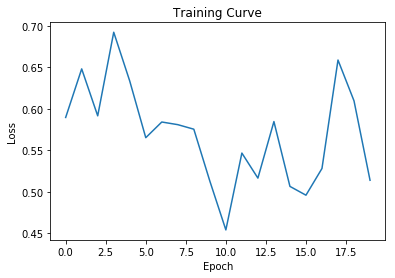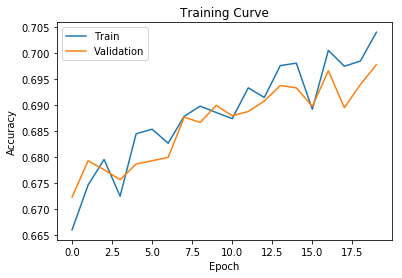Out:
0.700419248997448

The hidden size and the input embedding size don't have to be the same.

In :
#model = TweetRNN(50, 100, 2)


## LSTM for Long-Term Dependencies¶

There are variations of recurrent neural networks that are more powerful. One such variation is the Long Short-Term Memory (LSTM) module. An LSTM is like a more powerful version of an RNN that is better at perpetuating long-term dependencies. Instead of having only one hidden state, an LSTM keeps track of both a hidden state and a cell state.

In :
lstm_layer = nn.LSTM(input_size=50,   # dimension of the input repr
hidden_size=50,   # dimension of the hidden units
batch_first=True) # input format is [batch_size, seq_len, repr_dim]


Remember the single tweet that we worked with earlier?

In :
tweet_emb.shape

Out:
torch.Size([31, 50])

This is how we can feed this tweet into the LSTM, similar to what we tried with the RNN earlier.

In :
tweet_input = tweet_emb.unsqueeze(0) # add the batch_size dimension
h0 = torch.zeros(1, 1, 50)     # initial hidden layer
c0 = torch.zeros(1, 1, 50)     # initial cell state
out, last_hidden = lstm_layer(tweet_input, (h0, c0))
out.shape

Out:
torch.Size([1, 31, 50])

So an LSTM version of our model would look like this:

In :
class TweetLSTM(nn.Module):
def __init__(self, input_size, hidden_size, num_classes):
super(TweetLSTM, self).__init__()
self.emb = nn.Embedding.from_pretrained(glove.vectors)
self.hidden_size = hidden_size
self.rnn = nn.LSTM(input_size, hidden_size, batch_first=True)
self.fc = nn.Linear(hidden_size, num_classes)

def forward(self, x):
# Look up the embedding
x = self.emb(x)
# Set an initial hidden state and cell state
h0 = torch.zeros(1, x.size(0), self.hidden_size)
c0 = torch.zeros(1, x.size(0), self.hidden_size)
# Forward propagate the LSTM
out, _ = self.rnn(x, (h0, c0))
# Pass the output of the last time step to the classifier
out = self.fc(out[:, -1, :])
return out

model_lstm = TweetLSTM(50, 50, 2)

Epoch 1; Loss 0.595566; Train Acc 0.707189; Val Acc 0.701532
Epoch 2; Loss 0.568199; Train Acc 0.706937; Val Acc 0.700894
Epoch 3; Loss 0.432072; Train Acc 0.708165; Val Acc 0.701806
Epoch 4; Loss 0.529787; Train Acc 0.707441; Val Acc 0.701076
Epoch 5; Loss 0.557969; Train Acc 0.708543; Val Acc 0.702353
Epoch 6; Loss 0.590583; Train Acc 0.707724; Val Acc 0.701258
Epoch 7; Loss 0.475807; Train Acc 0.708323; Val Acc 0.700985
Epoch 8; Loss 0.612683; Train Acc 0.709173; Val Acc 0.702444
Epoch 9; Loss 0.660903; Train Acc 0.708701; Val Acc 0.700711
Epoch 10; Loss 0.613527; Train Acc 0.710843; Val Acc 0.701897
Epoch 11; Loss 0.587833; Train Acc 0.708953; Val Acc 0.700802
Epoch 12; Loss 0.503231; Train Acc 0.709551; Val Acc 0.701350
Epoch 13; Loss 0.446064; Train Acc 0.710433; Val Acc 0.702717
Epoch 14; Loss 0.660676; Train Acc 0.710969; Val Acc 0.702170
Epoch 15; Loss 0.548568; Train Acc 0.707346; Val Acc 0.699617
Epoch 16; Loss 0.563536; Train Acc 0.712072; Val Acc 0.701988
Epoch 17; Loss 0.553041; Train Acc 0.712261; Val Acc 0.702170
Epoch 18; Loss 0.584345; Train Acc 0.712702; Val Acc 0.702900
Epoch 19; Loss 0.559511; Train Acc 0.711946; Val Acc 0.703265
Epoch 20; Loss 0.516795; Train Acc 0.712765; Val Acc 0.703812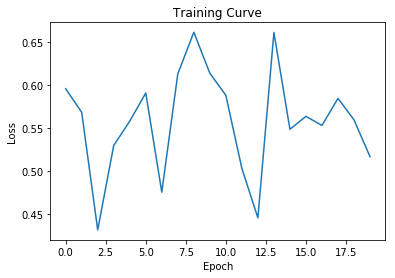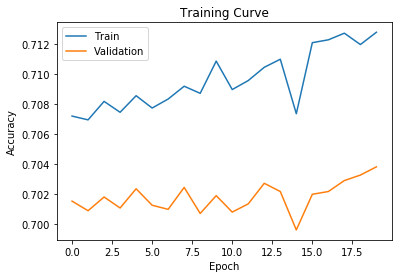Out:
0.7036091870215093

## GRU for Long-Term Dependencies¶

Another variation of an RNN is the Gated-Recurrent Unit (GRU). The GRU is invented after LSTM, and is intended to be a simplification of the LSTM that is still just as powerful. The nice thing about GRU units is that they have only one hidden state.

In :
gru_layer = nn.GRU(input_size=50,   # dimension of the input repr
hidden_size=50,   # dimension of the hidden units
batch_first=True) # input format is [batch_size, seq_len, repr_dim]


The GRU API is virtually identical to that of the vanilla RNN:

In :
tweet_input = tweet_emb.unsqueeze(0) # add the batch_size dimension
h0 = torch.zeros(1, 1, 50)     # initial hidden layer
out, last_hidden = gru_layer(tweet_input, h0)
out.shape

Out:
torch.Size([1, 31, 50])

So a GRU version of our model would look similar to before:

In :
class TweetGRU(nn.Module):
def __init__(self, input_size, hidden_size, num_classes):
super(TweetGRU, self).__init__()
self.emb = nn.Embedding.from_pretrained(glove.vectors)
self.hidden_size = hidden_size
self.rnn = nn.GRU(input_size, hidden_size, batch_first=True)
self.fc = nn.Linear(hidden_size, num_classes)

def forward(self, x):
# Look up the embedding
x = self.emb(x)
# Forward propagate the GRU
out, _ = self.rnn(x)
# Pass the output of the last time step to the classifier
out = self.fc(out[:, -1, :])
return out

model_lstm = TweetGRU(50, 50, 2)

Epoch 1; Loss 0.506148; Train Acc 0.712985; Val Acc 0.702444
Epoch 2; Loss 0.509147; Train Acc 0.711568; Val Acc 0.702900
Epoch 3; Loss 0.550659; Train Acc 0.713426; Val Acc 0.703812
Epoch 4; Loss 0.482575; Train Acc 0.713773; Val Acc 0.703082
Epoch 5; Loss 0.552732; Train Acc 0.713836; Val Acc 0.703447
Epoch 6; Loss 0.560482; Train Acc 0.714088; Val Acc 0.704997
Epoch 7; Loss 0.588303; Train Acc 0.712072; Val Acc 0.701806
Epoch 8; Loss 0.512033; Train Acc 0.715001; Val Acc 0.703812
Epoch 9; Loss 0.483773; Train Acc 0.714655; Val Acc 0.703994
Epoch 10; Loss 0.480774; Train Acc 0.715127; Val Acc 0.705180
Epoch 11; Loss 0.572385; Train Acc 0.712765; Val Acc 0.702444
Epoch 12; Loss 0.527220; Train Acc 0.715820; Val Acc 0.705818
Epoch 13; Loss 0.569705; Train Acc 0.717112; Val Acc 0.703903
Epoch 14; Loss 0.431541; Train Acc 0.716734; Val Acc 0.704906
Epoch 15; Loss 0.493773; Train Acc 0.715474; Val Acc 0.703173
Epoch 16; Loss 0.525030; Train Acc 0.716198; Val Acc 0.704906
Epoch 17; Loss 0.582700; Train Acc 0.717584; Val Acc 0.706639
Epoch 18; Loss 0.493774; Train Acc 0.717080; Val Acc 0.704633
Epoch 19; Loss 0.598182; Train Acc 0.715789; Val Acc 0.703356
Epoch 20; Loss 0.579206; Train Acc 0.717899; Val Acc 0.705818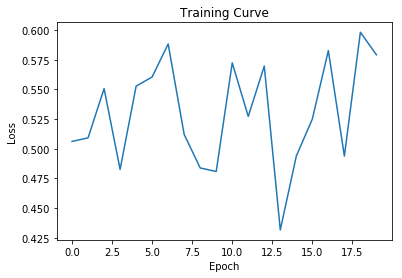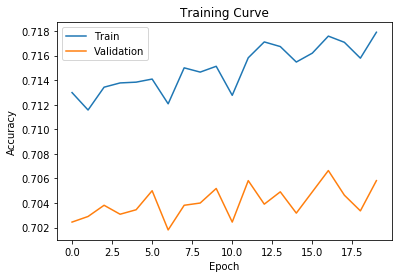Out:
0.7082573824279985